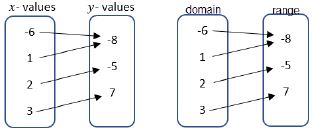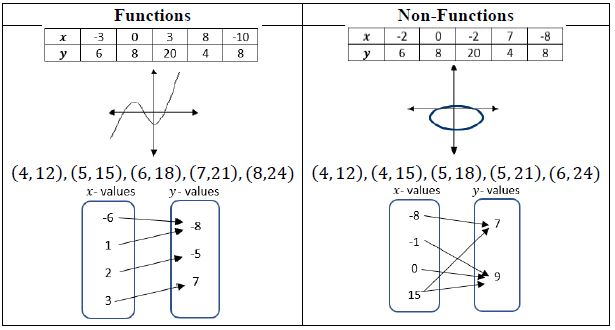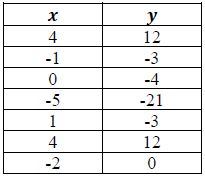# MA.8.F.1.1Export Print
Given a set of ordered pairs, a table, a graph or mapping diagram, determine whether the relationship is a function. Identify the domain and range of the relation.

### Clarifications

Clarification 1: Instruction includes referring to the input as the independent variable and the output as the dependent variable.

Clarification 2: Within this benchmark, it is the expectation to represent domain and range as a list of numbers or as an inequality.

General Information
Subject Area: Mathematics (B.E.S.T.)
Strand: Functions
Status: State Board Approved

## Benchmark Instructional Guide

• Domain
• Function
• Range

### Vertical Alignment

Previous Benchmarks

Next Benchmarks

### Purpose and Instructional Strategies

In grade 7, students determined whether two quantities have a proportional relationship by examining a table, graph or written description and they determined the constant of proportionality. In grade 8, students work with linear equations with two variables and begin the introduction of functions. In Algebra 1, students will classify the function type and represent it using function notation.
• A mapping diagram consists of a list of $x$-values and their corresponding $y$-values shown with an arrow.
• An example of a mapping diagram can include domain and range values or $x$- and $y$-values.• The “vertical line test” should be treated with caution because (1) it allows you to apply a rule without thinking and (2) it may create misconceptions for later mathematics.
• Vocabulary is important in this benchmark as it connects to future learning related to domain and range.
• Students should explain how they verified if the given context was a function or non-function (MTR.4.1). Students should provide counterexamples to deepen their knowledge of the relationships in functions.
• For example, students can be asked to create $x$- and $y$-values that create relations that are functions and non-functions.• Domain and range can be shown as a list, an inequality or as a verbal description depending on how the relation is given. The inequalities can be represented as inclusive or non-inclusive as determined by the context.
• For example, if a graph represents a real-world context, with non-negative values, with the equation $y$ = 6$x$ + 5, the domain and range can be described as below.
• List
A list cannot be used to represent this relation because it has infinitely many values.
• Inequality
Domain: $x$ ≥ 0; Range: $y$ ≥ 5
• Verbal Description
The domain is all real numbers that are greater than or equal to zero. The range is all real numbers that are greater than or equal to five.
• For example, for the relation {(4, 12), (5, 15), (6, 18), (7,21), (8,24)}, the domain and range can be described as below.
• List
Domain: {4, 5, 6, 7, 8}; Range: {12, 15, 18, 21, 24}
• Inequality
An inequality, such as 4 ≤ $x$ ≤ 8, cannot be used to represent this relation because it is based on a discrete set of values.
• Verbal Description
The domain is all whole numbers from four to eight, inclusive. The range is the multiples of three from 12 to 24, inclusive.

### Common Misconceptions or Errors

• Students may invert the terms independent and dependent variable. To address this misconception, focus on the vocabulary and relationship to the input and output.

### Strategies to Support Tiered Instruction

• Teacher reviews vocabulary and the difference between the terms. Once students understand that independent variables represent the input of the relation, they can make sense of real-world problems to accurately identify independent and dependent variables.
• For example, in a scientific experiment one can determine that input as the variable that is controlled by the scientist. So the independent variable is the one that is controlled in the experiment and the dependent is the result of the experiment.
• Teacher creates a matching activity with real-world situations. Students match dependent variable and the independent variable for the situation. Teacher facilitates discussion among students on their reasoning behind their matches from the activity in order to clear up any lingering misconceptions.
• Instruction includes helping students see how no number within the domain is repeated when the relationship is a function.

A relation is shown below where $x$ represents the independent variable and $y$ represents the dependent variable.
(3, 4), (−2, 3), (7, 1), (−$\frac{\text{1}}{\text{2}}$ , 4) , (−2, $\frac{\text{1}}{\text{2}}$)
• Part A. Create a mapping diagram, table and graph to represent this relation.
• Part B. Determine the domain and range of the relation.
• Part C. Determine if the relation represents a function or does not represent a function and justify your decision.
• Part D. If the relation is not a function, which point could be removed to make it a function? If it is a function, add a point that would no longer make it a function.

### Instructional Items

Instructional Item 1
A relation is shown in the table below where $x$ represents the independent variable and ?? represents the dependent variable. Decide whether the table can represent a function or cannot represent a function.Instructional Item 2
Identify the domain and range for the relation {(3, 8), (2, 3), (1, 0), (0, −1), (−1, 0)}.

*The strategies, tasks and items included in the B1G-M are examples and should not be considered comprehensive.

## Related Courses

This benchmark is part of these courses.
1205050: M/J Accelerated Mathematics Grade 7 (Specifically in versions: 2014 - 2015, 2015 - 2020, 2020 - 2022, 2022 and beyond (current))
1205070: M/J Grade 8 Pre-Algebra (Specifically in versions: 2014 - 2015, 2015 - 2022, 2022 and beyond (current))
1204000: M/J Foundational Skills in Mathematics 6-8 (Specifically in versions: 2014 - 2015, 2015 - 2022, 2022 and beyond (current))
7812030: Access M/J Grade 8 Pre-Algebra (Specifically in versions: 2014 - 2015, 2015 - 2018, 2018 - 2019, 2019 - 2022, 2022 and beyond (current))

## Related Access Points

Alternate version of this benchmark for students with significant cognitive disabilities.
MA.8.F.1.AP.1a: Given a set of ordered pairs, a table or mapping diagram identify whether the relationship is a function.
MA.8.F.1.AP.1b: Given a set of ordered pairs, a table or mapping diagram identify the domain and range of the relation.

## Related Resources

Vetted resources educators can use to teach the concepts and skills in this benchmark.

## Formative Assessments

Which Sequences Are Functions?:

Students are asked to determine if each of two sequences is a function and to describe its domain, if it is a function.

Type: Formative Assessment

Recognizing Functions:

Students are asked to determine whether or not each of two graphs represent functions.

Type: Formative Assessment

What Is a Function?:

Students are asked to define the term function and describe any important properties of functions.

Type: Formative Assessment

Car Wash:

Students are asked to describe the domain of a function given its graph.

Type: Formative Assessment

Circles and Functions:

Students are shown the graph of a circle and asked to identify a portion of the graph that could be removed so that the remaining portion represents a function.

Type: Formative Assessment

Cafeteria Function:

Students are asked decide if one variable is a function of the other in the context of a real-world problem.

Type: Formative Assessment

Identifying Functions:

Students are asked to determine if relations given by tables and mapping diagrams are functions.

Type: Formative Assessment

Identifying the Graphs of Functions:

Students are given four graphs and asked to identify which represent functions and to justify their choices.

Type: Formative Assessment

What Is a Function?:

Students are asked to define the term function including important properties.

Type: Formative Assessment

Tabulating Functions:

Students are asked to determine whether or not tables of ordered pairs represent functions.

Type: Formative Assessment

Identifying Algebraic Functions:

Students are asked to determine if each of three equations represents a function. Although the task provides equations, in their explanations students can use other representations such as ordered pairs, tables of values or graphs.

Type: Formative Assessment

## Lesson Plan

Functions: Domain and Range:

Students will identify if a graph represents a function and determine domain and range of the graphs.

Type: Lesson Plan

## Original Student Tutorials

Functions, Functions Everywhere: Part 1:

What is a function? Where do we see functions in real life? Explore these questions and more using different contexts in this interactive tutorial.

This is part 1 in a two-part series on functions. Click HERE to open Part 2.

Type: Original Student Tutorial

Driven By Functions:

Learn how to determine if a relationship is a function in this interactive tutorial that shows you inputs, outputs, equations, graphs and verbal descriptions.

Type: Original Student Tutorial

## Tutorials

Dependent and Independent Variables Exercise:

In an equation with 2 variables, we will be able to determine which is the dependent variable, and which is the independent variable.

Type: Tutorial

Vertical Line Test:

A graph in Cartesian coordinates may represent a function or may only represent a binary relation. The "vertical line test" is a visual way to determine whether or not a graph represents a function.

Type: Tutorial

## Video/Audio/Animations

Real-Valued Functions of a Real Variable:

Although the domain and codomain of functions can consist of any type of objects, the most common functions encountered in Algebra are real-valued functions of a real variable, whose domain and codomain are the set of real numbers, R.

Type: Video/Audio/Animation

Domain and Range of Binary Relations:

Two sets which are often of primary interest when studying binary relations are the domain and range of the relation.

Type: Video/Audio/Animation

## MFAS Formative Assessments

Cafeteria Function:

Students are asked decide if one variable is a function of the other in the context of a real-world problem.

Car Wash:

Students are asked to describe the domain of a function given its graph.

Circles and Functions:

Students are shown the graph of a circle and asked to identify a portion of the graph that could be removed so that the remaining portion represents a function.

Identifying Algebraic Functions:

Students are asked to determine if each of three equations represents a function. Although the task provides equations, in their explanations students can use other representations such as ordered pairs, tables of values or graphs.

Identifying Functions:

Students are asked to determine if relations given by tables and mapping diagrams are functions.

Identifying the Graphs of Functions:

Students are given four graphs and asked to identify which represent functions and to justify their choices.

Recognizing Functions:

Students are asked to determine whether or not each of two graphs represent functions.

Tabulating Functions:

Students are asked to determine whether or not tables of ordered pairs represent functions.

What Is a Function?:

Students are asked to define the term function including important properties.

What Is a Function?:

Students are asked to define the term function and describe any important properties of functions.

Which Sequences Are Functions?:

Students are asked to determine if each of two sequences is a function and to describe its domain, if it is a function.

## Original Student Tutorials Mathematics - Grades 6-8

Driven By Functions:

Learn how to determine if a relationship is a function in this interactive tutorial that shows you inputs, outputs, equations, graphs and verbal descriptions.

## Original Student Tutorials Mathematics - Grades 9-12

Functions, Functions Everywhere: Part 1:

What is a function? Where do we see functions in real life? Explore these questions and more using different contexts in this interactive tutorial.

This is part 1 in a two-part series on functions. Click HERE to open Part 2.

## Student Resources

Vetted resources students can use to learn the concepts and skills in this benchmark.

## Original Student Tutorials

Functions, Functions Everywhere: Part 1:

What is a function? Where do we see functions in real life? Explore these questions and more using different contexts in this interactive tutorial.

This is part 1 in a two-part series on functions. Click HERE to open Part 2.

Type: Original Student Tutorial

Driven By Functions:

Learn how to determine if a relationship is a function in this interactive tutorial that shows you inputs, outputs, equations, graphs and verbal descriptions.

Type: Original Student Tutorial

## Tutorials

Dependent and Independent Variables Exercise:

In an equation with 2 variables, we will be able to determine which is the dependent variable, and which is the independent variable.

Type: Tutorial

Vertical Line Test:

A graph in Cartesian coordinates may represent a function or may only represent a binary relation. The "vertical line test" is a visual way to determine whether or not a graph represents a function.

Type: Tutorial

## Video/Audio/Animations

Real-Valued Functions of a Real Variable:

Although the domain and codomain of functions can consist of any type of objects, the most common functions encountered in Algebra are real-valued functions of a real variable, whose domain and codomain are the set of real numbers, R.

Type: Video/Audio/Animation

Domain and Range of Binary Relations:

Two sets which are often of primary interest when studying binary relations are the domain and range of the relation.

Type: Video/Audio/Animation

## Parent Resources

Vetted resources caregivers can use to help students learn the concepts and skills in this benchmark.